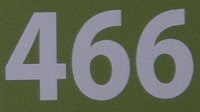## Monday, June 15, 2009

### 466

466 = 2 x 233. It is a semiprime that is the product of two distinct Sophie Germain primes.

Each digit of 466 is a semiprime.

466 is 1234 in base 7.

466 has a representation as a sum of two squares: 466 = 52 + 212.

466 arises in the sequence created by starting with 1 and repeatedly reversing the digits and adding five: 1, 6, 11, 16, 66, 71, . . . , 164, 466, 669, . . .466 is the maximum number of regions into which 30 lines divide a plane.

Source: Positive Integers